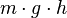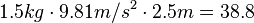# Gravitational energy facts for kids

Kids Encyclopedia Facts

Gravitational energy is the potential energy held by an object because of its high position compared to a lower position. In other words, it is energy associated with gravity or gravitational force. For example, a pen being held above a table has a higher gravitational potential than a pen sitting on the table. Gravitational potential energy is mechanical energy minus kinetic energy. It has a scalar quantity measured in Joules (J).

An object gets gravitational potential energy when it moves uphill. The energy used up when moving up the hill is changed into gravitational potential energy. When the object comes back down again, the gravitational potential energy is turned back into kinetic energy (movement). That is why riding a bike up a steep hill is very hard, but when you are coming back down, you will not need to pedal at all - you are being powered by the gravitational potential energy that you stored when you rode up the hill. Another way gravitational potential energy helps us is by keeping earth and the other planets in orbit in our solar system around the Sun.

## Formula and example

The mathematical formula for the gravitational potential energy:

Gravitational potential energy =$m\cdot g \cdot h$

Where:

Most scientists and students use these measurement unit:

For example, an object with a mass of 1.5 kilograms that is 2.5 metres above the ground would have a gravitational potential energy value of:$1.5 kg \cdot 9.81 m/s^2 \cdot 2.5 m = 38.8$ joules.Gravitational energy Facts for Kids. Kiddle Encyclopedia.